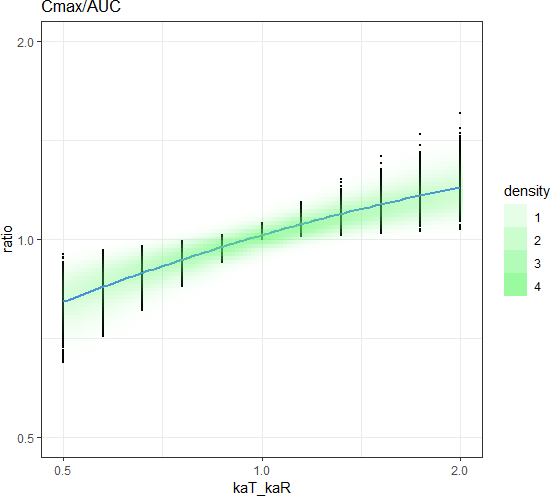## Conclusion & beyond [NCA / SHAM]

Hi mittyri,

» » I corrected a typo in your original post
»
» forgot to execute it on clean environment, sorry!

Welcome to the club! BTW, THX for implementing the lin-up/log-down trapezoidal.» » So what do you conclude?
»
» pAUC is more sensitive, but the sensitivity is still low (nothing new...)

Yep. Actually this story reaches too far (for an IR formulation crossing flip-flop PK; no regulator would buy that regardless what is written in a guideline) and not far enough: Setting the cut-off for pAUC at the individual tmax-values of the reference is Canadian tradition (termed AUCReftmax) but even in Canada history. If pAUCs are used, the cut-off has to be pre-specified. Currently pAUCs are not required for IR. OK, if the first pAUC performs better than Cmax, great.
I think that limiting ka(T)/ka(R) to 0.5–2 is sufficient.
For the cut-off 2×tmax=2log(ka/ke/(ka–ke)), what else?

Edit: No wonder you hated this line of your code. Shouldn’t it be:
SubjectsDFstack <-   reshape(SubjectsDF[, -c(2,3,4,6,7,9,11)],     direction = 'long', varying = c(3:5), v.names = "ratio",     timevar = "metric", times = names(SubjectsDF)[c(7,9,11)])

My sim’s: In the input section t.cut <- 2*log(ka/(CL/Vd)/(ka-(CL/Vd)))
Then (relevant lines only):
AbsorptionDF <- function(D, ka, Vd, CL,t,ratio,t.cut){   # Reference   ke       <- CL/Vd   C        <- C.sd(D=D, Vd=Vd, ka=ka, ke=ke, t=t)   tmax     <- t[C == max(C)]   Cmax     <- C.sd(D=D, Vd=Vd, ka=ka, ke=ke, t=tmax)   AUC.t    <- AUCcalc(t, C)   t.1      <- t[which(t <= t.cut)]   C.1      <- C[which(t <= t.cut)]   pAUC     <- AUCcalc(t.1, C.1)   Cmax.AUC <- Cmax/AUC.t   DF.sub <- cbind(Subject = isub, V = Vd.sub, CL = CL.sub,                   AbsorptionDF(D, ka.sub, Vd.sub, CL.sub, t, ratio, t.cut)) sp1 <- ggplot(SubjectsDFstack[SubjectsDFstack$metric == "Cmax", ], aes(x=kaT_kaR, y=ratio, color=factor(metric))) sp1 + theme_bw() + geom_point(size=.3) + geom_smooth(method = 'loess', se = FALSE) + stat_density_2d(data = SubjectsDFstack[SubjectsDFstack$metric == "Cmax", ],     geom = "raster", aes(alpha = ..density..), fill = "#F8766D",     contour = FALSE) +   scale_alpha(range = c(0, 0.7)) +   scale_x_continuous(trans='log2') +   scale_y_continuous(limits=c(0.5,2), trans='log2') sp2 <- ggplot(SubjectsDFstack[SubjectsDFstack$metric == "pAUC", ], aes(x=kaT_kaR, y=ratio, color=factor(metric))) sp2 + theme_bw() + geom_point(size=.3) + geom_smooth(method = 'loess', se = FALSE) + stat_density_2d(data = SubjectsDFstack[SubjectsDFstack$metric == "pAUC", ],     geom = "raster", aes(alpha = ..density..), fill = "#6DAAF8",     contour = FALSE) +   scale_alpha(range = c(0, 0.7)) +   scale_x_continuous(trans='log2') +   scale_y_continuous(limits=c(0.5,2), trans='log2') sp3 <- ggplot(SubjectsDFstack[SubjectsDFstack$metric == "Cmax_AUC", ], aes(x=kaT_kaR, y=ratio, color=factor(metric))) sp3 + theme_bw() + geom_point(size=.3) + geom_smooth(method = 'loess', se = FALSE) + stat_density_2d(data = SubjectsDFstack[SubjectsDFstack$metric == "Cmax_AUC", ],     geom = "raster", aes(alpha = ..density..), fill = "#6DF876",     contour = FALSE) +   scale_alpha(range = c(0, 0.7)) +   scale_x_continuous(trans='log2') +   scale_y_continuous(limits=c(0.5,2), trans='log2')Based on loess:
   metric kaT_kaR predicted sensitivity  Cmax_AUC     0.5 0.8082842  0.54181715      Cmax     0.5 0.8075275  0.54298163      pAUC     0.5 0.7143879  0.76337666      pAUC     2.0 1.2181904  0.08875421  Cmax_AUC     2.0 1.2012353  0.10671423      Cmax     2.0 1.2028693  0.10745301

Again, pAUC is the one-eyed leading the blind ones but only if kaT < kaR.

Dif-tor heh smusma 🖖
Helmut SchützThe quality of responses received is directly proportional to the quality of the question asked. 🚮
Science QuotesIng. Helmut Schütz